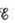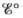Chapter 18, Problem 71E

Chapter
Section
Textbook Problem

# The overall reaction in the lead storage battery is Pb ( s )   +  PbO 2 ( s )   +  2H + ( a q ) +  2HSO 4 − ( a q )       → 2PbSO 4 ( s )   +  2H 2 O ( l ) Calculateat 25°C for this battery when [H2SO4] = 4.5 M, that is, [H+] = [HSO4−] = 4.5 M. At 25°C,=2.04 V for the lead storage battery.

Interpretation Introduction

Interpretation:

The overall reaction taking place in the lead storage battery is given. The value of E at 25°C for this battery at the given concentrations of different ions is to be calculated.

Concept introduction:

The relationship between reduction potential and standard reduction potential value and activities of species present in an electrochemical cell at a given temperature is given by the Nernst equation.

The value of Ecell is calculated using Nernst formula,

E=E°(RTnF)ln(Q)

At room temperature the above equation is specifies as,

E=E°(0.0591n)log(Q)

To determine: The value of E at 25°C for this battery at the given concentrations of different ions.

Explanation

Given

The value of concentration of H+ is 0.45M .

The value of concentration of HSO4 is 0.45M .

The value of E°cell is 2.04V .

The reaction taking place in a lead storage battery is given as,

Pb(s)+PbO2(s)+2H+(aq)+2HSO4(aq)2PbSO4(s)+2H2O(l)

The reaction taking place at cathode is,

PbO2(s)+HSO4(aq)+3H+(aq)+2ePbSO4(s)+2H2O(l)

The reaction taking place at anode is,

Pb(s)+HSO4(aq)PbSO4(s)+H+(aq)+2e

The reaction involves the transfer of 2 moles of electrons and the value of E°cell=2

### Still sussing out bartleby?

Check out a sample textbook solution.

See a sample solution

#### The Solution to Your Study Problems

Bartleby provides explanations to thousands of textbook problems written by our experts, many with advanced degrees!

Get Started